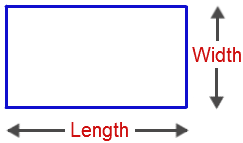# Perimeter of a Rectangle Formula

In this article, we will discuss the perimeter of a rectangle in detail along with its rectangle perimeter formula and a few solved examples. We know that the perimeter of any two-dimensional shape is equal to the total distance covered by the boundary of the given shape.

## Perimeter of a Rectangle

The perimeter of a rectangle is defined as the sum of all the sides of a rectangle. For any polygon, the perimeter formulas are the total distance around its sides. In case of a rectangle, the opposite sides of a rectangle are equal and so, the perimeter will be twice the width of the rectangle plus twice the height of the rectangle and it is denoted by the alphabet “p”## Perimeter of a Rectangle Formula

The perimeter of rectangle formula is given by

The perimeter of a rectangle, p = 2 ( l+b) units

where

“l” is the length of the rectangle

“b” is the breadth of the rectangle

### Solved Examples

Example 1: Find the perimeter of a rectangle whose length is 9 cm and width is 16 cm.

Solution:

Given,

Length = 9 cm

Width = 16 cm

Perimeter of Rectangle= 2(Length + Breadth)

= 2(9 + 16) cm

= 2 x 25 cm

Therefore, the perimeter of a rectangle= 50 cm

Example 2: Find the perimeter of a rectangle whose length and width is 20 cm and 9 cm respectively.

Solution:

Given,

Length = 20 cm

Width = 9 cm

Perimeter of Rectangle = 2(Length + Breadth)

= 2(20 +9) cm

= 2 x 29 cm

Therefore, the perimeter of a rectangle = 58 cm

For more math formulas and concepts, keep visiting BYJU’S. Also, register at BYJU’S to get complete assistance for the maths preparation and get various video on mathematics related topics explained in an easy and engaging way.

 Related Links Area of a Circle Area of Rectangle Perimeter of Square Perimeter of Triangle